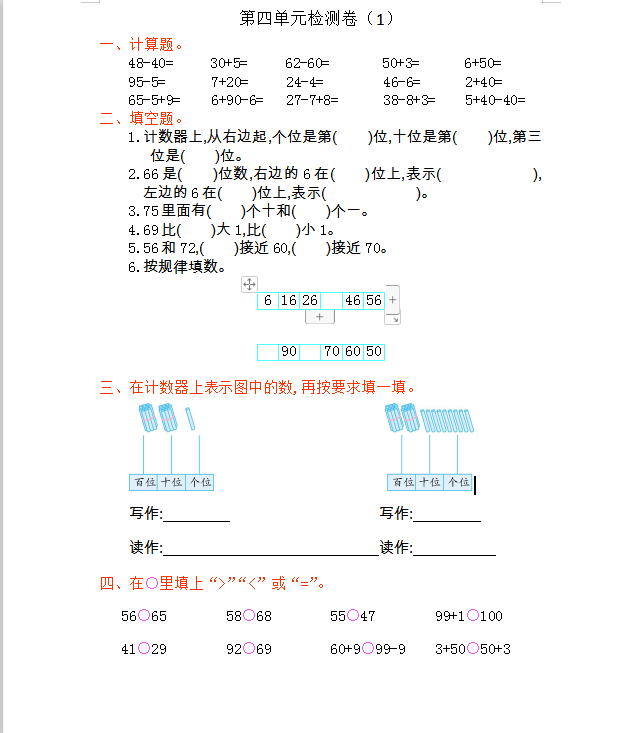48-40=　 　30+5=　 　62-60=　 　　50+3=　　　   6+50=

95-5=             7+20=         24-4=                 46-6=               2+40=

65-5+9=        6+90-6=     27-7+8=             38-8+3=           5+40-40=

1.计数器上,从右边起,个位是第(　　)位,十位是第(　　)位,第三位是(　　)位。

2.66是(　　)位数,右边的6在(　　)位上,表示(　　　　　　),左边的6在(　　)位上,表示(　　　　　　)。

3.75里面有(　　)个十和(　　)个一。

4.69比(　　)大1,比(　　)小1。

5.56和72,(　　)接近60,(　　)接近70。

6.按规律填数。